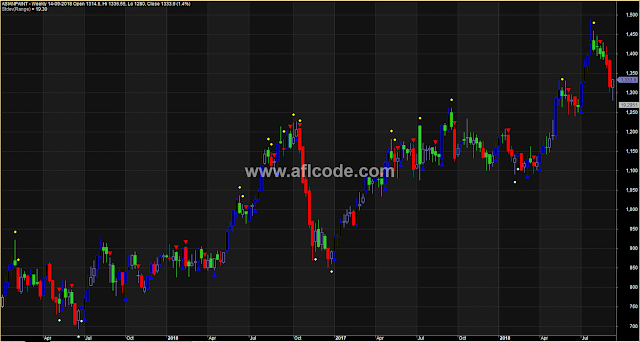### Colorful Candles With Buy Sell IndicationColorful Candles With Buy Sell Indication

```//www.aflcode.com
_SECTION_BEGIN("Aneks_Colors");
SetChartOptions(0,chartShowArrows|chartShowDates);
_N(Title = StrFormat("{{NAME}} - {{INTERVAL}} {{DATE}} Open %g, Hi %g, Lo %g, Close %g (%.1f%%) {{VALUES}}", O, H, L, C, SelectedValue( ROC( C, 1 ) ) ));

UP_Mild = colorBlueGrey;
UP_Strong = colorBlue;
DN_Mild = colorLime;
DN_Strong = colorRed;
Doji  = colorLightGrey;

paint = IIf(C > O, IIf(C > Ref(H,-1), UP_Strong, UP_Mild), Doji);
paint = IIf(C < O, IIf(C < Ref(L,-1), DN_Strong, DN_Mild), paint);

Plot(C, "Close", paint,  styleNoTitle | GetPriceStyle());

_SECTION_END();

_SECTION_BEGIN("UP_Thrust");
//UP Thrust

Cycle_Length = Param("Cycle_Length_Bars", 10, 2,30,1);
Range = High - Low;

TPB  = Volume > MA(Volume,9) AND Close < Low + Range/2 AND Range <= Ref(Range,-1); //Topping Bar
UTB  = Volume < MA(Volume,9) AND Close > Low + Range/2; //Upthrust Bar

//Setup01 = TPB ;
//Setup02 = UTB AND (  Ref(TPB,-1) OR Ref(TPB,-2) OR Ref(TPB,-3) OR Ref(TPB,-4) OR Ref(TPB,-5) OR Ref(TPB,-6) OR Ref(TPB,-7) OR Ref(TPB,-8) OR Ref(TPB,-9) OR Ref(TPB,-10) );
Setup03 = HHV(H,3) > Ref(HHV(H,20),-2) AND C < (HHV(H,2) + LLV(L,2))/2;
Setup04 = LLV(L,3) < Ref(LLV(L,20),-2) AND C > (HHV(H,2) + LLV(L,2))/2;

PlotShapes(shapeSmallCircle * Setup03,colorYellow,0,H,20);
PlotShapes(shapeSmallCircle * Setup04,colorSkyblue,0,L,-20);

TR = StDev(range,BarCount-100);
Plot(TR,"\nStdev(Range)",colorLightGrey, styleNoLine | styleOwnScale);

_SECTION_BEGIN("DiNapoli's Trend");

EMA_Diff  = EMA( Close, 8.3896) - EMA( Close, 17.5185);
Cond03  = EMA_Diff  >= Ref(EMA_Diff,-1);
Cond01  = Close >= Ref(MA(Close,3),-2);

//Cond02 , Not coded yet

UP   = Cond01 AND Cond03 ;
DN  = NOT Cond01 AND NOT Cond03;

UP   = ExRem(UP,DN);
DN   = ExRem(DN,UP);

PlotShapes(shapeSmallUpTriangle * UP, colorBlue, 0, L);
PlotShapes(shapeSmallDownTriangle * DN, colorRed, 0, H);
_SECTION_END();

_SECTION_END();
```

Previous
Next Post »